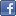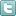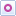EE Times-India > Sensors/MEMS

Sensors/MEMS# Utilising FETS for sensor applications (Part 2)

Posted: 10 Jul 2015Print Version

Keywords:op amps  FET  photodiode preamp  transresistance amplifiers  hydrophone

A low noise JFET such as an LSK189 is configured as a source follower, with a source biasing resistor R3. In terms of input capacitance at the gate of J1 with an LSK189, it is about 3 pF from the gate to the drain, which is much less than the 18 pF of Cin(-). Capacitance between the gate and ground due to the gate to source capacitance approaches zero. This is because the source follower configuration provides substantially the same AC voltage at the gate and at the source, which substantially cancels out the capacitance between the gate and the source. The source follower also greatly reduces the capacitance seen at the gate to ground even when the source is driving signal into a capacitive load, Cin(-), the capacitance at the (-) input terminal of the op amp U1A.

It should be noted that the source follower circuit may add some phase shift to the overall amplifier circuit. To ensure phase margin and no oscillations, a resistive divider R1 and R2 is used. With the values given at 3900Ω for R1 and R2, the equivalent feedback resistance is [1 + (R2/R1)] x RF = 2 x 1MΩ = 2MΩ, the same resistance value shown in figure 8 for RF.

If the (+) input of the op amp in figure 9 is grounded, such that Voffset = 0V, Vout will most likely have a DC offset. To "zero" Vout when there is no signal from the photodiode, a clean DC voltage, Voffset may be applied to the (+) input of U1.

Op amp U1A = AD797 has an equivalent input noise voltage of 0.9 nV/√Hz and J1 =LSK189 with an equivalent input noise voltage of 1.8 nV/√Hz, the total equivalent input noise voltage is about 2.0 nV/√Hz. This is lower than a very low noise JFET op amp such as an AD743 that has 3.2 nV/√Hz.

Note that bipolar input stage op amps AD797 with 0.9 nV/√Hz has lower equivalent input noise voltage than 2.0 nV/√Hz, but the AD797's input noise current is too high and are not suitable for amplifier circuits with large value feedback resistors (RF) in the MΩ such as the circuit shown in Figure 8.

Note that J1 may be substituted with an LSK170 (0.9nV/√Hz ) if a slight increase in capacitance from the gate to ground is acceptable. This FET has about half the equivalent input noise of the LSK189.

Extending the frequency response of a transresistance amplifier

In transresistance amplifiers, a feedback resistor will have a capacitance, CF, across its leads. This capacitance will reduce the bandwidth of the output signal at high frequencies. See figures 10(a) and 10(b).Figure 10: (a) Simple preamp; (b) simple preamp with equalisation.

Figure 10(a) shows a photodiode preamp with Ct = Cpd + Cin(-), and CF a capacitor across the feedback resistor RF. CF can be the internal parasitic capacitance from resistor RF, or it can be capacitor often connected across RF to add positive phase shift that offsets the negative shift from Ct. This added positive phase shift due to CF ensures stability in the photodiode preamp. However, one side effect from CF is reducing the bandwidth of the amplified photodiode signal at Vout.

The -3 dB bandwidth at Vout is 1/[2π(RF)(CF)] = f-3dB

For example, RF = 2MΩ and CF = 1pF, then f-3dB = 1/[2π(2MΩ)( 1pF)] = 79.6kHz

One way to reduce the effects this roll off in frequency response is to make RF a series connection of ten 200KΩ resistors. If CF is still 1pF, the new bandwidth will be:

f-3dB = 1/[2π(200KΩ)( 1pF)] = 796kHz

However, there is another alternative and that is to equalise the frequency response as shown in figure 10(b).

For now, let's assume that that op amp U1A in figure10(b) has sufficient phase margin to not oscillate when RA = 0Ω, and when RB = open circuit or removed.

If (R1 + R2)(C3) = (RF)(CF) there will be a "boost" that compensates for the roll-off. When the boost ends is determined by the (R2)(C2) time constant. The amount of bandwidth extension is [1+ (R1/R2)]. Generally there is a limit on bandwidth extension. If R2→0Ω, then C3 is grounded and forms a low pass filter and lagging phase shift network with R1, that will most likely cause oscillation.

Generally, it is recommended to start with R2 = 33% of R1, which gives a bandwidth extension of [1 + (R1/0.33R1)] = [1 + 3] = 4 = bandwidth extension.

For example, if RF= 2MΩ and CF = 1pF, we want R1 <C3 = (2MΩ)(1pF)/(13.3KΩ) = 150 pf = C3. With these values, the frequency response will increase from 79.6kHz to about 318kHz for Figure 10(b).

Note: Often the op amp U1A may require voltage divider resistors RA and RB to ensure sufficient phase margin so that the amplifier in figure10(b) does not oscillate. Typically, start out with a voltage divider of 2 or more. That is, (RA/RB) ≥ 1. Also, it is recommended that RA||RB ≤ 500Ω and that RA||RB << R1.

An equalised preamplifier
Figure 11 shows an equalised preamplifier with discrete FETs at the input terminals.

 Related Articles Editor's Choice
Comment on "Utilising FETS for sensor applicatio..."

Top Ranked Articles

WebinarsVisit Asia Webinars to learn about the latest in technology and get practical design tips.

Search EE Times India
Services

Go to topConnect on FacebookFollow us on TwitterFollow us on Orkut
﻿

Back to Top